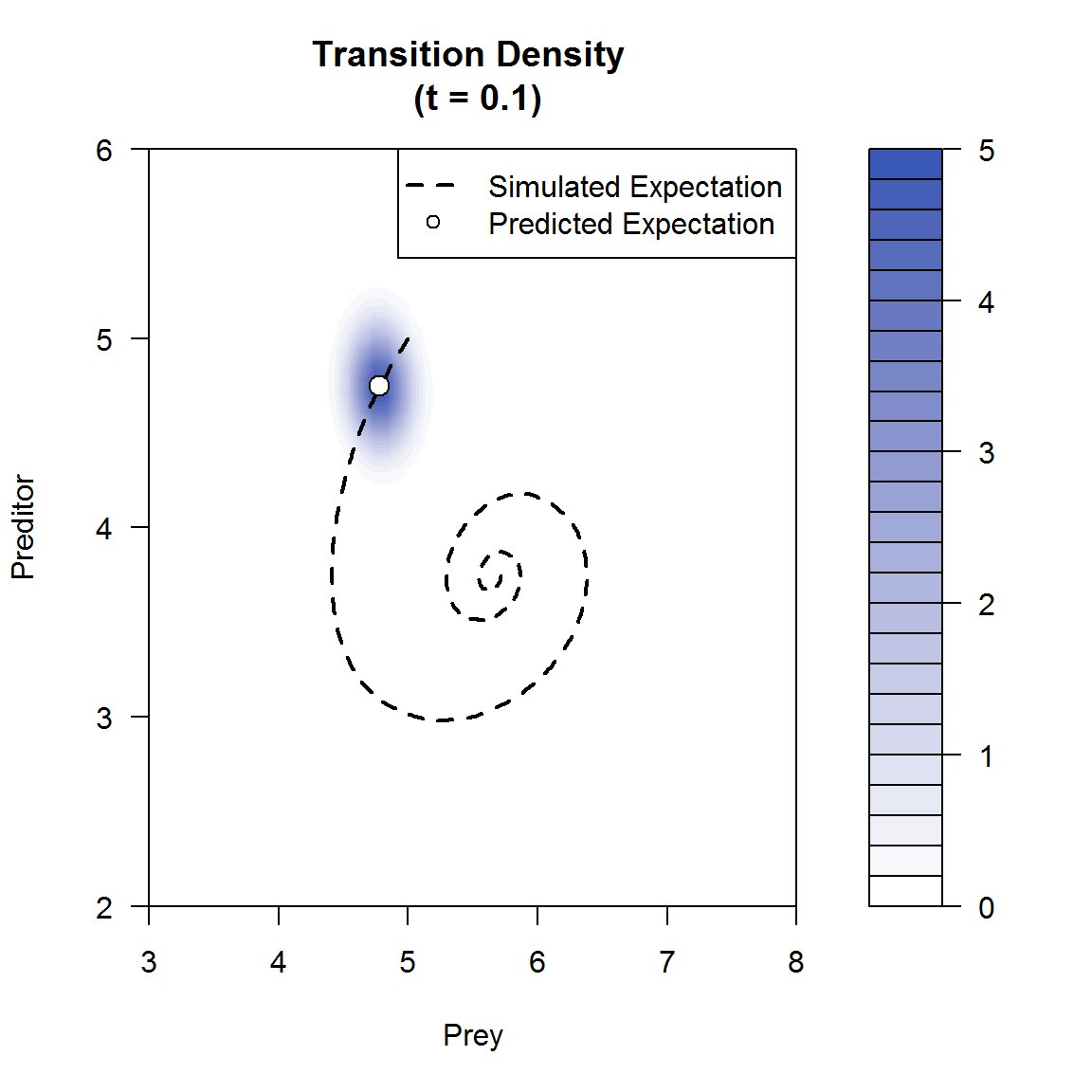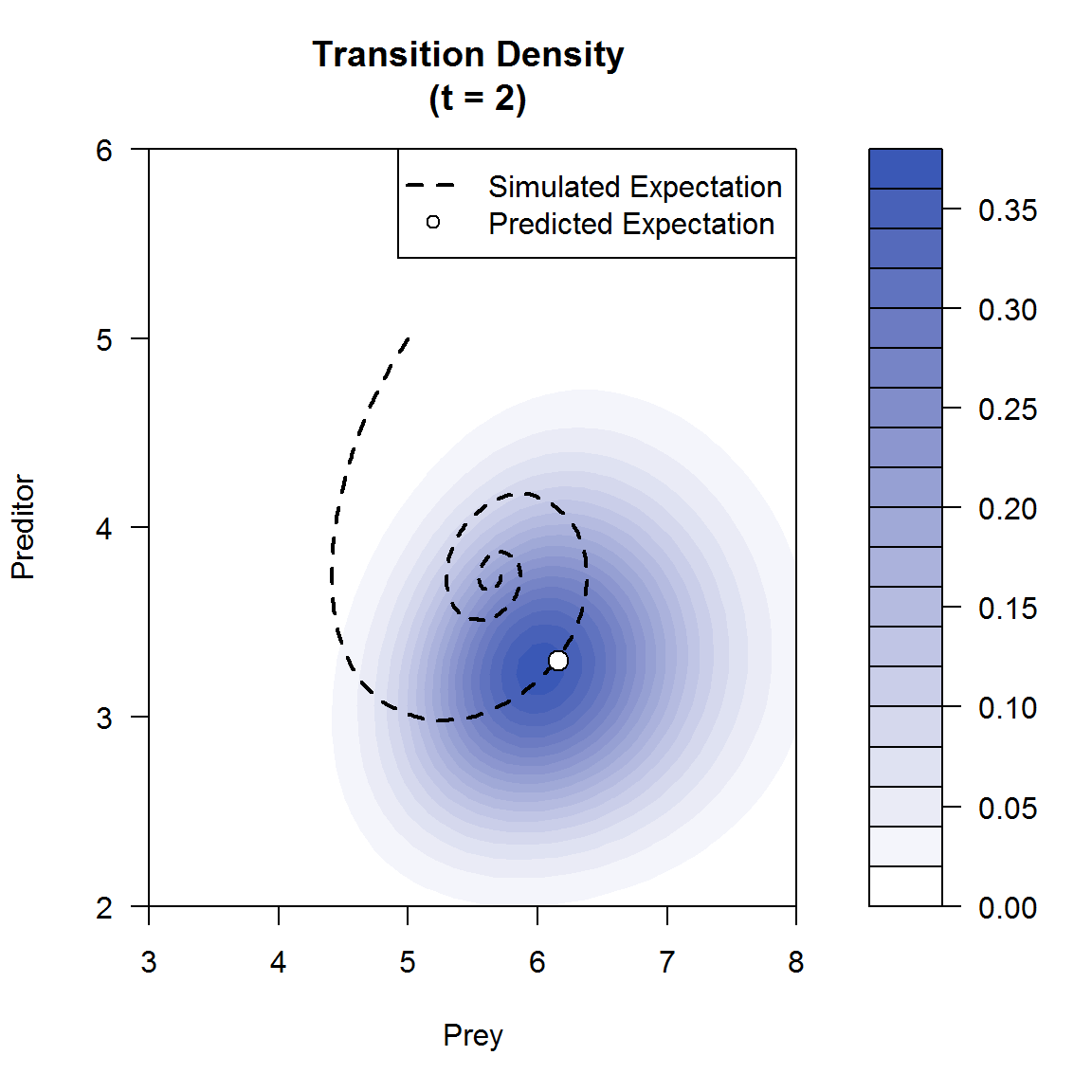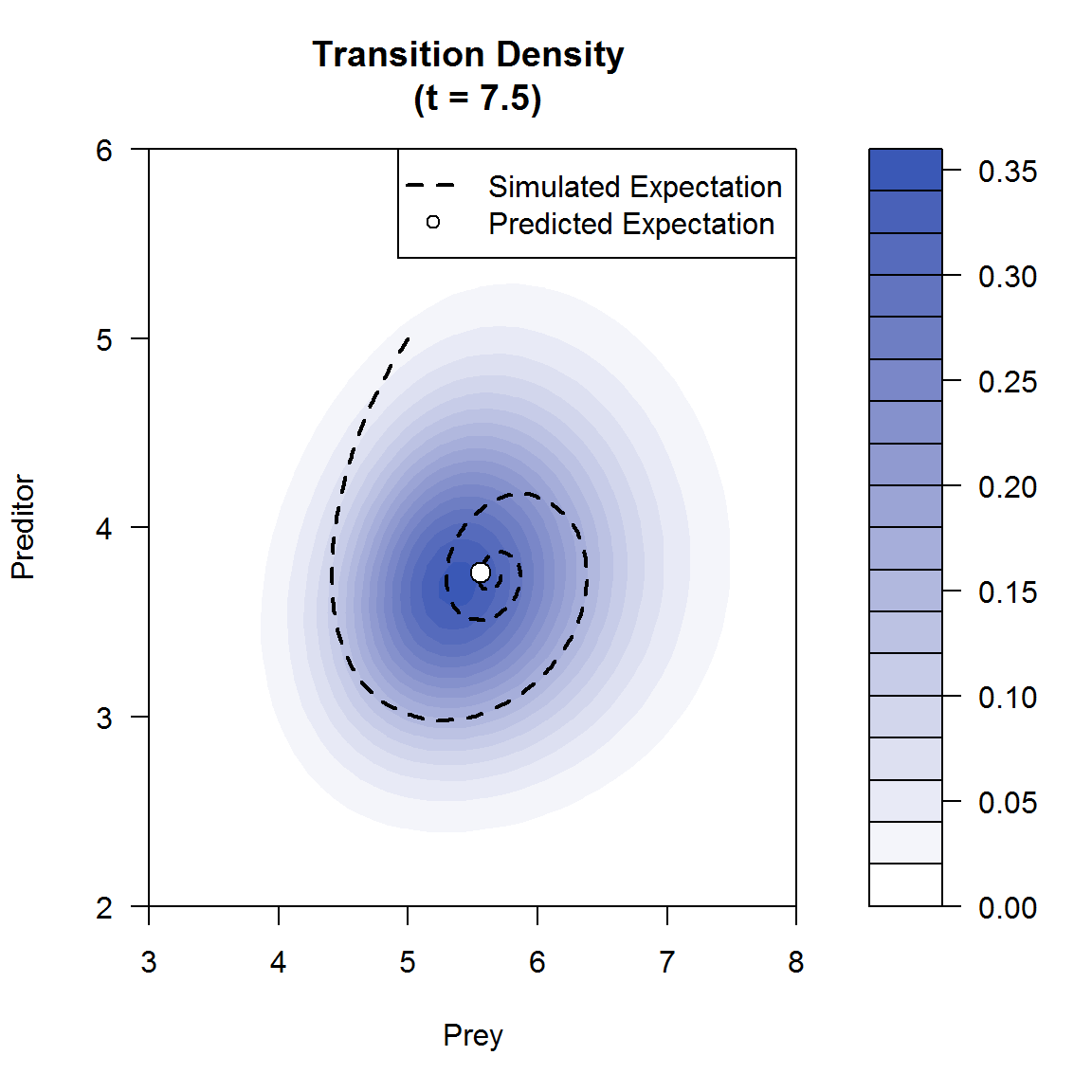# Cumulant truncation procedure

DiffusionRgqd approximates the solution to the Kolmogorov forward eqn $\frac{\partial}{\partial t} f(X_t|X_s) = -\frac{\partial}{\partial X_t} [\mu(X_t,t) f(X_t|X_s)]+\frac{1}{2}\frac{\partial^2}{\partial X_t^2} [\sigma^2(X_t,t) f(X_t|X_s)],$ by calculating the conditional moment trajectories of a diffusion process over time and subsequently carrying these moments into a suitable surrogate density. For example, given a scalar generalized quadratic diffusion:

$dX_t = (G_0(t)+G_1(t)X_t+G_2(t)X_t^2)dt +\sqrt{Q_0(t)+Q_1(t)X_t+Q_2(t)X_t^2}dB_t,$

it is possible to derive a system of differential equations that govern the evolution of the cumulants of the process over time. Let $$\kappa_i(t)$$ denote the $$i$$-th cumulant of $$X_t$$ given that the process assumes the initial value $$X_s$$, then: \begin{aligned} \frac{\partial}{\partial t}{\kappa}_j(t)=&G_0(t)\mbox{Ind}(j = 1)\\ &+G_1(t) j\kappa_{j}(t)\\ &+G_2(t)\biggl(j\kappa_{j+1}(t)+\sum_{r=1}^{j}r{j\choose r}\kappa_{r}(t)\kappa_{j-r+1}(t)\biggr)\\ &+Q_0(t)\mbox{Ind}(j = 2)\\ &+Q_1(t)\frac{j(j-1)}{2}\kappa_{j-1}(t)\mbox{Ind}(j \geq 2)\\ &+Q_2(t)\biggl(\frac{j(j-1)}{2}\kappa_j(t)+\frac{j}{2}\sum_{r=1}^{j-1}r {j-1\choose r} \kappa_{r}(t)\kappa_{j-1-r+1}(t)\biggr)\mbox{Ind}(j \geq 2),\\ \end{aligned} for $$i = 1,2,\ldots$$. By solving these equations numerically using high order Runge-Kutta methods, we may plug the resulting moment trajectories into a suitable surrogate density such as a Normal distribution or a saddlepoint approximation in order to approximate the transitional density.

# Generate the transition density of a time-inhomogeneous GQD

As an introductory example to the DiffusionRgqd package we approximate the transitional density of a scalar diffusion over an arbitrarily chosen transition horizon. This can be achieved using the GQD.density() function. GQD.density() generates the transitional density of a GQD for a given initial value using the cumulant truncation procedure developed by (Varughese 2013). The function serves as a good starting point for any analysis being conducted using the DiffusionRgqd package as it allows one to check whether a proposed model does not exhibit nonsensical dynamics with respect to the problem at hand. Perhaps more importantly, it can be used to check the validity of the density approximation and/or an appropriate truncation order used for the cumulants.

Consider a Cox-Ingersoll-Ross (CIR) (Cox, Ingersoll Jr, and Ross 1985) process with time-dependent coefficients:

$dX_t = 2(10+\sin(2\pi (t-0.5))-X_t)dt+\sqrt{0.25(1+0.75\sin(4 \pi t))X_t}dB_t.$

In order to analyse this SDE in R we need to define the model within the workspace. Since DiffusionRgqd uses a functional input interface (see the introductory vignette) which relies on declaring the functional form of a given model’s coefficients, we need to make sure that the workspace doesn’t contain any object names that might clash with those of the model coefficients. We can do this by simply running the GQD.remove() command:

 library(DiffusionRgqd)
GQD.remove()
##  "Removed :  NA "

If any objects are recognized with names that may clash with names reserved for use with the {GQD.density()} function they will subsequently be removed. In this case we used a vanilla {R} session so the function will simply indicate that no clashes were detected and removed. The purpose of the {GQD.remove()} function is to act as a model-eraser in situations where multiple models are being defined in a single R session. The next step is to write the SDE in terms of its coefficients. We can define the SDE in the current R session by declaring the coefficient functions:

 G0 <- function(t){2*(10+sin(2*pi*(t-0.5)))}
G1 <- function(t){-2}
Q1 <- function(t){0.25*(1+0.75*(sin(4*pi*t)))}

The functions {G0}, {G1} and {Q1} together constitute the R-language counterpart of the SDE under the generalized quadratic framework. Note that for scalar GQDs we have capitalized the coefficient names in order to avoid the possibility of calling {q()} accidentally, in which case the R console will prompt to quit.

In order to approximate the transitional density using the cumulant truncation procedure we need to define the peripheral parameters of the problem. This consists of giving the initial condition of the SDE (the starting value of the diffusion), the starting time of the diffusion and the geometry of the transitional horizon, i.e. spatial values where the density is to be evaluated and the corresponding time mesh. These elements can be defined by assigning values to the arguments of GQD.density(): Xs, Xt, s, t and delt. Respectively, these parameters represent the initial value, the values at which to evaluate the transition density, the starting time, the final time and the step size for the time mesh. That is, for a diffusion process starting at time s in state Xs, the transition density is approximated at times s+delt, s+2*delt … up to and including t at all values of the vector Xt. In R:

 states    <- seq(5, 15, 1/10)
initial   <- 8
Tmax      <- 5
Tstart    <- 1
increment <- 1/100

M <- GQD.density(Xs = initial, Xt = states, s = Tstart, t = Tmax, delt = increment)
##
##  ================================================================
##  ================================================================
##  _____________________ Drift Coefficients _______________________
##  G0 : 2*(10+sin(2*pi*(t-0.5)))
##  G1 : -2
##  G2
##  ___________________ Diffusion Coefficients _____________________
##  Q0
##  Q1 : 0.25*(1+0.75*(sin(4*pi*t)))
##  Q2
##  __________________ Distribution Approximant ____________________
##  P               :
##  alpha           :
##  Trunc. Order    : 4
##  Dens.  Order    : 4
## =================================================================

GQD.density() creates a list containing a matrix of density values, spatial coordinates at which the density was evaluated, corresponding time coordinates for the time mesh and finally a matrix of trajectories for the cumulants and moments that were used in evaluating the density approximation. In this case we have assigned the output to an object called M for further use in the workspace. Since the density approximation is evaluated over a space-time lattice it can best be visualized with a perspective plot:

persp(x = M$Xt, y = M$time, z = M$density, col = 'white', xlab = 'State (X_t)', ylab = 'Time (t)', zlab = 'Density f(X_t|X_s)', border = NA, shade = 0.5, theta = 145)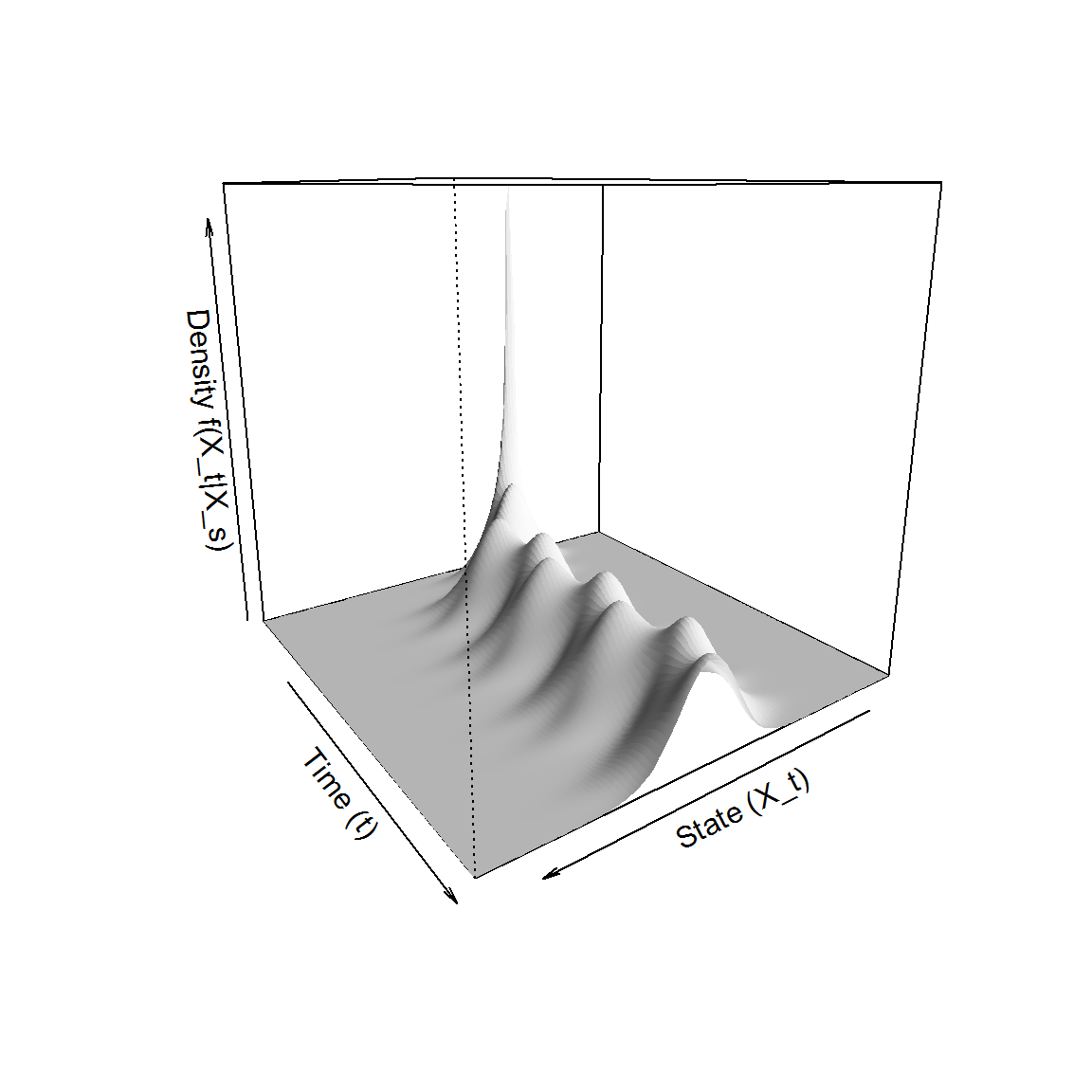Alternatively, one can simply use GQD.plot(M) in order to visualize a given density approximation in a similar way. Note that we have not directly specified what truncation order to be used in the calculation of the density approximation. The default is to use a 4-th order truncation in conjunction with a 4-th order truncated saddlepoint approximation. In most cases it suffices to use the default settings although, as will be shown in the following example, little effort is required to extend the analysis to higher order truncations and/or alternate density approximations. Although this example operates well within the limits of the capabilities of the methodology it serves to illustrate the simplicity of the interface of the DiffusionRgqd package - often producing desired results in less than 10 lines of code with minimal mathematical input. # Time-inhomogeneous Jacobi diffusion: A scalar diffusion with finite support Although most practical applications of diffusion processes result in the use of diffusions with uni-modal transitional densities it is still possible to conceive of a diffusion within the GQD framework that exhibits atypical dynamics. For example, a particularly interesting phenomena occurs when considering GQDs for which the diffusion term is of the form: $\sigma(X_t,t) = c\sqrt{X_t(1-X_t)}.$ Assuming the process starts within the interval $$[0,1]$$, whenever the diffusion approaches the bounds $$0$$ or $$1$$, the dynamics of the process is dominated by the behaviour of the drift function, $$\mu(X_t,t)$$. Provided $$\partial/{\partial x} \mu(x,t)|_{x=0}>0, \partial/{\partial x} \mu(x,t)|_{x=1}<0 \quad\forall t$$ are sufficiently large, the process will reflect from the boundaries and remain within the interval $$[0,1]$$. A special case of this behaviour can be seen with the so-called Jacobi diffusion (Gouriéroux and Valéry 2004), whereby $$\mu(X_t,t)$$ is linear in $$X_t$$. For purposes of the illustration we shall assume a time-inhomogeneous Jacobi diffusion: $dX_t = a(b(1+0.25\sin(2\pi t))-X_t)dt+c\sqrt{X_t(1-X_t)}dB_t,$ with $$X_0,b \in [0,1]$$ and $$a>0$$. The diffusion exhibits oscillating drift dynamics whereby the process is pulled towards a point fluctuating between $$0.75b$$ and $$1.25b$$. If and when the trajectory of the process hits $$0$$ or $$1$$ it is reflected toward the interior of the domain. By applying the cumulant truncation procedure to the Jacobi diffusion we approximate the evolution of the cumulants for various orders of truncation under the Beta-type density from the multi-modal Pearson system (Cobb, Koppstein, and Chen 1983). This may be achieved in R using: # Set some parameter values: a <- 0.5; b <- 0.6; cc <- 1; X0 <- 0.5; # Give the coefficient form of the diffusion.: G0 <- function(t){a*(b*(1 + 0.25*sin(pi*t)))} G1 <- function(t){-a} Q1 <- function(t){cc} Q2 <- function(t){-cc} # What states should the density be evaluated at: states=seq(1/1000,1-1/1000,1/1000) # Generate the transitional density for truncation orders 4,6 and 8: res1 <- GQD.density(X0, states, 0, 2, 0.01, Dtype = 'Beta', Trunc = c(4,4)) ## ## ================================================================ ## Generalized Quadratic Diffusion (GQD) ## ================================================================ ## _____________________ Drift Coefficients _______________________ ## G0 : a*(b*(1+0.25*sin(pi*t))) ## G1 : -a ## G2 ## ___________________ Diffusion Coefficients _____________________ ## Q0 ## Q1 : cc ## Q2 : -cc ## __________________ Distribution Approximant ____________________ ## Density approx. : Beta ## P : 100 ## alpha : 0 ## Trunc. Order : 4 ## Dens. Order : 4 ## ================================================================= res2 <- GQD.density(X0, states, 0, 2, 0.01, Dtype = 'Beta', Trunc = c(6,6), print.output = FALSE) ## ================================================================= res3 <- GQD.density(X0, states, 0, 2, 0.01, Dtype = 'Beta', Trunc = c(8,8), print.output = FALSE) ## ================================================================= The objects res1, res2 and res3 now contain the transitional density approximations for truncation order 4, 6 and 8. The additional parameter Dtype = 'Beta' sets the density approximation to the multi-modal Beta class developed by Cobb, Koppstein, and Chen (1983). That is, let: $\mathbf{A} = \begin{pmatrix} 1&u_1(t)&\ldots&u_{m/2}(t)\\ u_1(t)&u_2(t)&\ldots& u_{m/2+1}(t)\\ \vdots&\vdots&\ddots&\vdots\\ u_{m/2}(t)&u_{m/2+1}(t)&\ldots&u_{m}(t)\\ \end{pmatrix},$ and $$\boldsymbol{\beta}=\{\beta_1,\beta_2,\ldots,\beta_{m/2+1}\}$$. Then the multi-modal Beta distribution of order $$m$$ is given by: $\tilde{f}_B^{(m)}(x|X_s) \propto x^{-\beta_1} (1-x)^{-\sum_{i=1}^{m/2+1}\beta_i}\exp\biggl(-\sum_{i=1}^{m/2-1}\bigl(\sum_{j=i+1}^{m/2}\beta_j\bigr)x^{i}/i\biggr) \quad \forall x \in [0,1],$ where $$\boldsymbol{\beta} = \mathbf{A}\mathbf{v}$$ and $$\mathbf{v}=\{v_i=iu_{i-1}(t)-(i+1)u_{i}(t):i=1,...,m/2+1\}^{\prime}$$. while the variable Trunc = c(6,6) sets the cumulant truncation (first item in c(6,6)) to $$m=6$$ (thus evaluating the cumulant equations for all orders $$i = 1, 2, \ldots, 6$$) whilst setting $$m=6$$ for the density approximation (second item in c(6,6)). Note that we separate the cumulant truncation order from that of the density in order to allow lower order density truncations under a given cumulant truncation order, for example Trunc=c(6,4). In order to check the accuracy of the approximation we may compare the approximate transition density to a simulated transition density of the Jacobi diffusion. This can be achieved by simulating a number of sample paths for the Jacobi diffusion and subsequently plotting a histogram of the resulting trajectories. For a diffusion process with SDE: $dX_t=\mu(X_t,t)dt+\sigma(X_t,t)dB_t$ we may simulate a trajectory at discrete time points by applying a Euler-Maruyama scheme to the Jacobi diffusion and evaluate the resulting difference equation iteratively. That is given an initial value $$X_{t_0}$$, we evaluate for all $$i=1,2,\ldots$$: $X_{t_i}=X_{t_{i-1}}+\mu(X_{t_{i-1}},t_{i-1})\Delta+\sigma(X_{t_{i-1}},t_{i-1}) r_{\Delta}$ where $$r_{\Delta}\sim \mbox{N}(0,\sigma^2=\Delta)$$ and $$t_i-t_{i-1}=\Delta$$. By plugging the drift and diffusion terms of the Jacobi diffusion into this updating equation we may simulate a number of trajectories for the restricted diffusion. However, since the Euler scheme is not exact the simulated paths may actually exit the $$[0,1]$$ region. As such we employ the simple modification that at each iteration $$X_{t_i}$$ is set to $$\min(\max(0,X_{t_{i-1}}+\mu(X_{t_{i-1}},t_{i-1})\Delta+\sigma(X_{t_{i-1}},t_{i-1}) r_{\Delta}),1)$$. By simulating a large number of trajectories we can compare each respective density approximation by superimposing the approximation over a histogram of the simulated trajectories at any given time point. This can easily be achieved using the code: N <- 100000 # Number of trajectories to simulate. smdelt <- 1/1000 # Simulation stepsize. d <- 0 # A variable for tracking time. X <- rep(X0,N) # Take snapshots of the density at these times: when <- c(0,0.25,0.5,1,1.75) for(i in 2:(2/smdelt)) { # A Euler-Maruyama type approximation: X <- pmax(pmin(X + (G0(d)+G1(d)*X)*smdelt+sqrt(Q1(d)*X+Q2(d)*X^2)*rnorm(length(X),sd=sqrt(smdelt)),1),0) d <- d+smdelt if(any(when==round(d,3))) { index <- which(res1$time==round(d,3))
hist(X, col='grey75', freq = FALSE, breaks = 30, ylim = c(0,3), border = 'white',
main = paste0('Transitional Density at t = ',round(d,3)))
lines(res1$density[,index]~res1$Xt, col = 'black'  , lty = 'dotted',lwd = 1)
lines(res2$density[,index]~res2$Xt, col = '#BBCCEE', lty = 'solid' ,lwd = 2)
lines(res3$density[,index]~res3$Xt, col = '#222299', lty = 'dashed',lwd = 2)
legend('top', legend=c('Truncation: (4,4).','Truncation: (6,6).',
'Truncation: (8,8).'), col = c('black','#BBCCEE','#222299'),
lty=c('dotted', 'solid', 'dashed'),lwd =c(1, 2, 2),bg='white')
}
}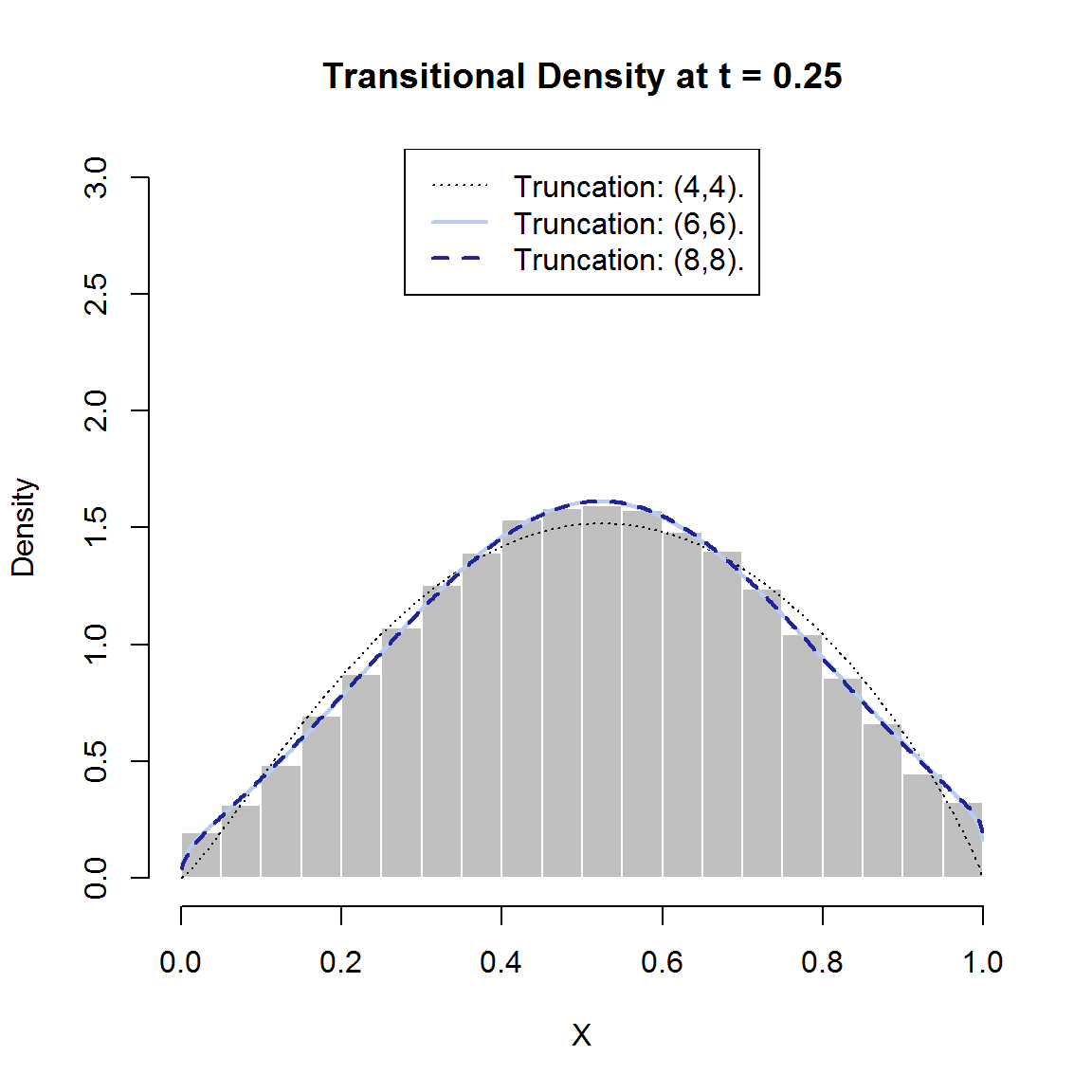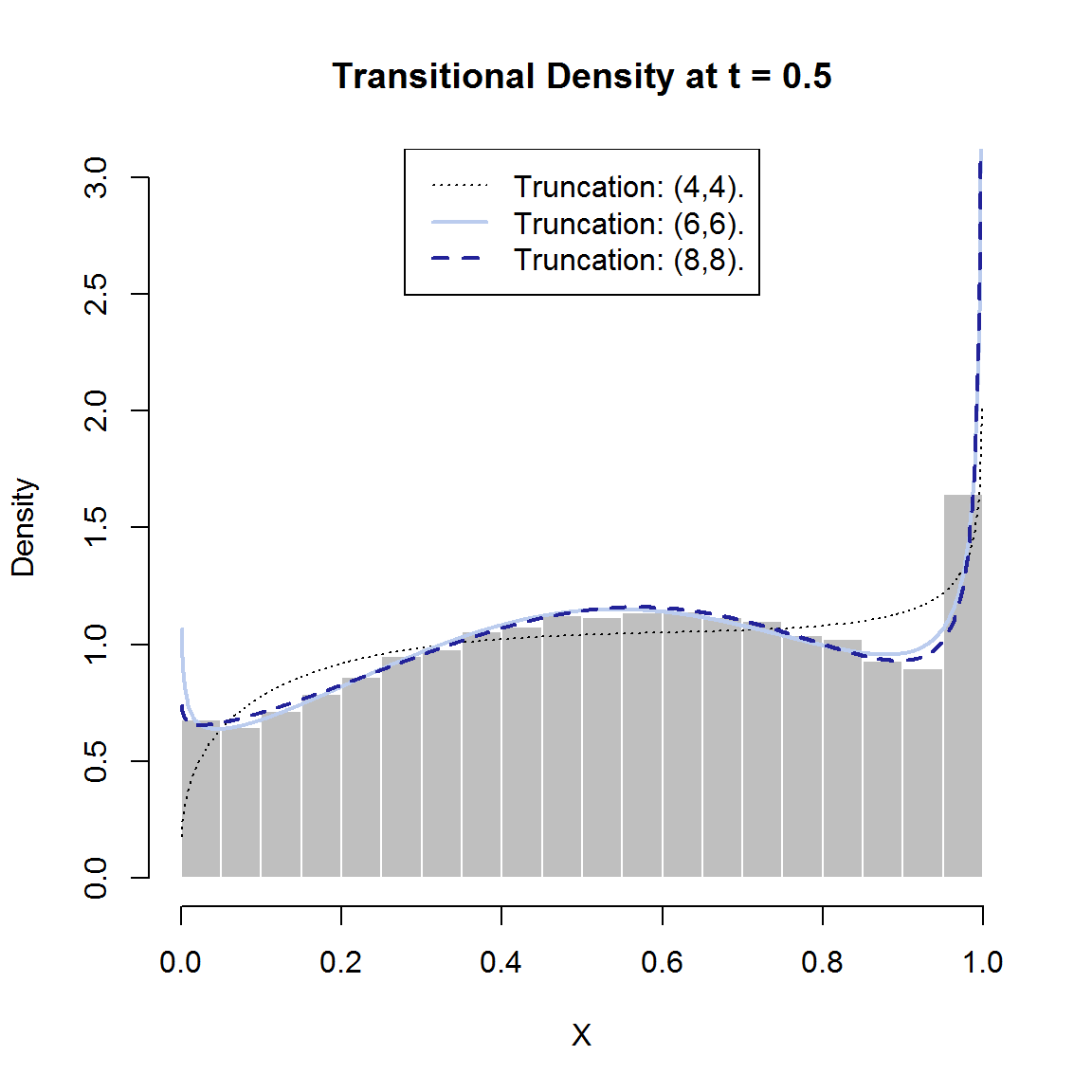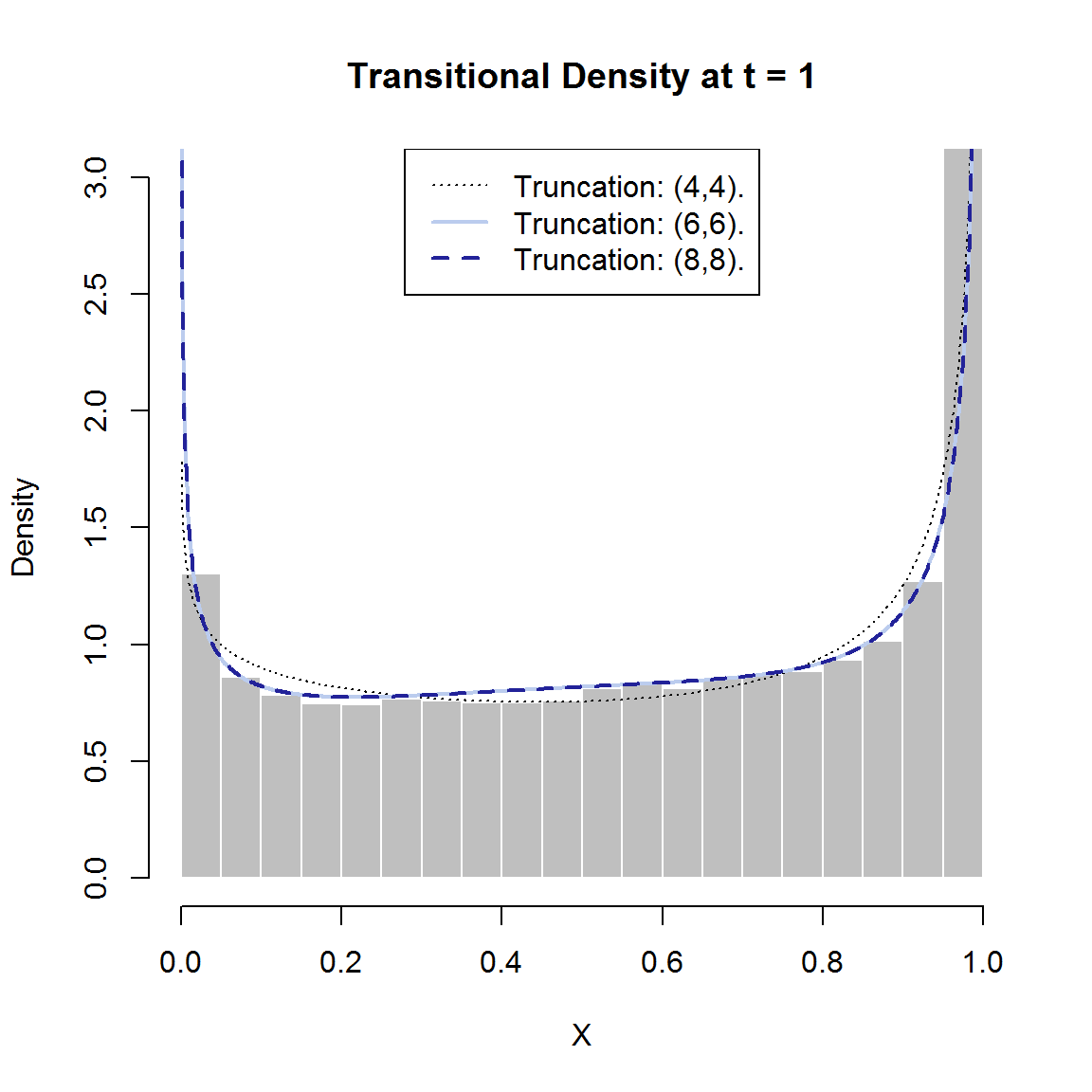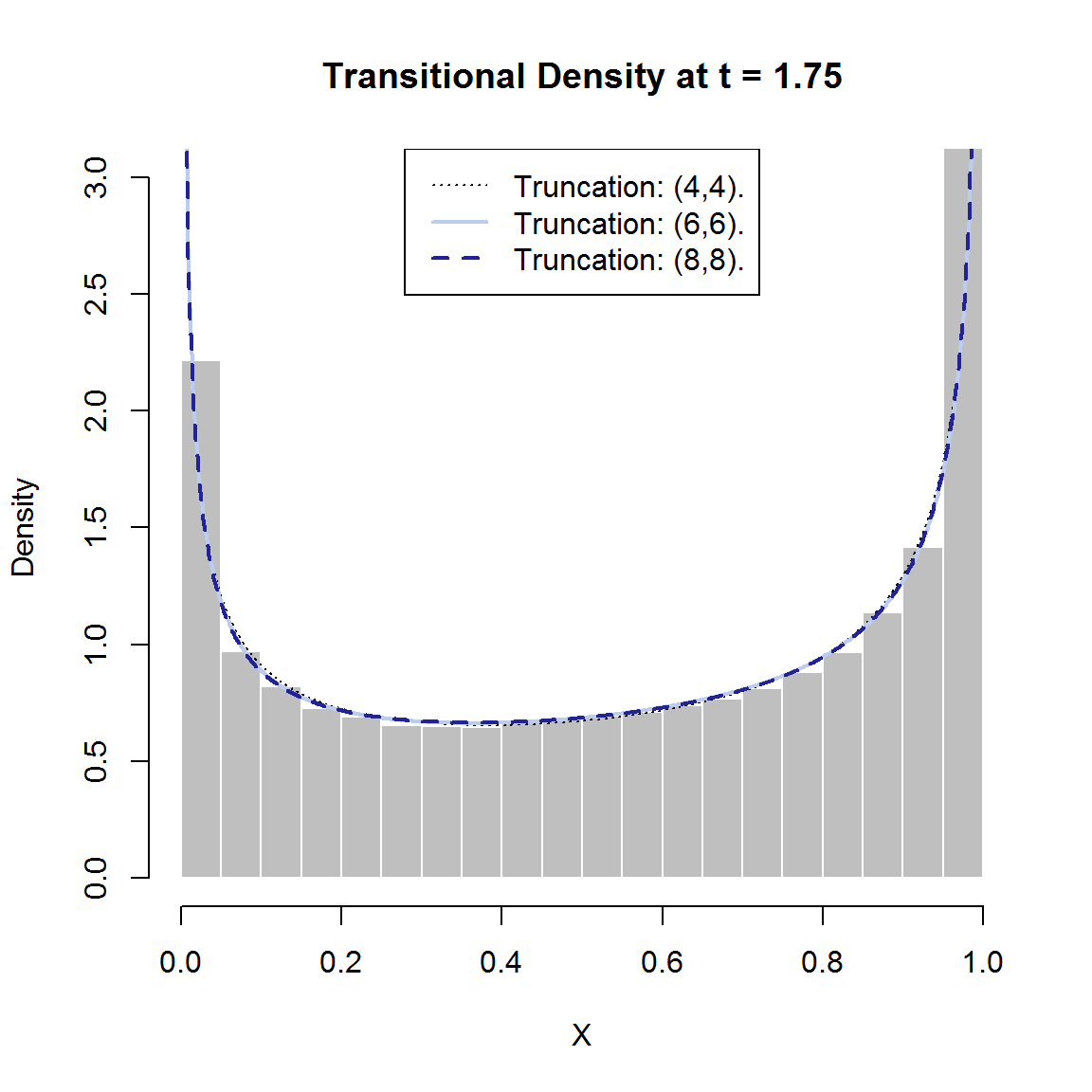The resulting figures suggest that the approximation remains accurate for truncation orders $$6$$ and $$8$$ whilst $$m=4$$ produces less desirable results. Interestingly, as time progresses it seems that all orders of approximation produce a reasonable approximation of the transition density. This may be due to the long-run dynamics of the process being somewhat simpler than in early phases in the transition horizon.

Using the persp() function we can again generate a perspective plot of the transition density in order to get an overall view of the transitional density. Using the $$m=6$$-th order truncation:

persp(x = res2$Xt, y = res2$time, z = res2density, col = 'white', xlab = 'State (X_t)', ylab = 'Time (t)', zlab = 'Density f(X_t|X_s)', border = NA, shade = 0.5, theta = 145)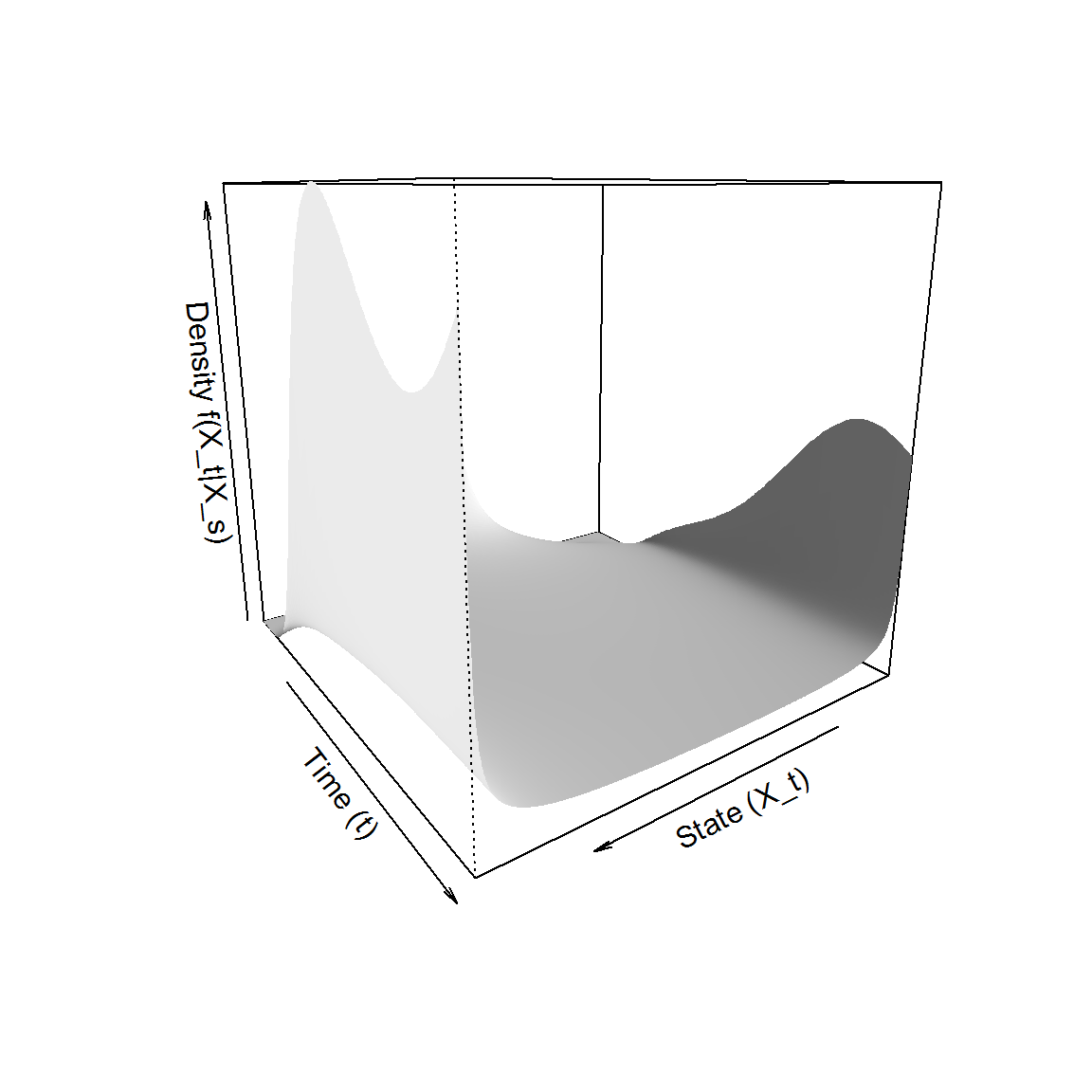As in the previous example this can be done by using the GQD.plot() command i.e., GQD.plot(res3). As is typical for diffusion processes we see that the transitional density starts out as an infinite point mass and shortly exhibits a normal-like distribution. As time progresses, however, the density transits into a U-shape’ with oscillating peakedness in conjunction with the sinusoidal term of the drift function. Although the DiffusionRgqd package allows one to perform comprehensive analysis on scalar quadratic diffusions, similar analysis can be conducted for interesting bivariate diffusions. Under the generalized quadratic framework the corresponding template stochastic differential equation is given by the system: $d\mathbf{X}_t =\boldsymbol\mu(\mathbf{X}_t,t)dt+\boldsymbol\sigma(\mathbf{X}_t,t)d\mathbf{B}_t$ where $\boldsymbol\mu(\mathbf{X}_t,t)= \begin{bmatrix} \sum_{i+j\leq 2}a_{ij}(t)X_t^iY_t^j\\ \sum_{i+j\leq 2}b_{ij}(t)X_t^iY_t^j\\ \end{bmatrix}$ and $\boldsymbol\sigma(\mathbf{X}_t,t)\boldsymbol\sigma^{\prime}(\mathbf{X}_t,t) = \begin{bmatrix} \sum_{i+j\leq 2}c_{ij}(t)X_t^iY_t^j&\sum_{i+j\leq 2}d_{ij}(t)X_t^iY_t^j\\ \sum_{i+j\leq 2}e_{ij}(t)X_t^iY_t^j&\sum_{i+j\leq 2}f_{ij}(t)X_t^iY_t^j\\ \end{bmatrix}.$ A model that is often used to illustrate non-linear dynamics in the analysis of ordinary differential equations is that of the Lotka-Volterra model. The corresponding ODEs are typically given as: \begin{aligned} \frac{\partial x_t}{\partial t} &= a x_t-bx_t y_t\\ \frac{\partial y_t }{\partial t} &= -c y_t+dx_t y_t\\ \end{aligned} for some positive $$a$$, $$b$$, $$c$$ and $$d$$. The equations are often used to describe the dynamics of two interacting populations wherein the population growth rate of the populations are mutually influenced by the current level of the opposing population. As such the model has been used to explain oscillatory behaviour in predator-prey relationships {Hoppensteadt2006} where $$x_t$$ denotes the prey population and $$y_t$$ the predator population at time $$t$$. Continuing with the predator-prey metaphor, perhaps one deficiency of the model, one might argue, is the absence of random input and subsequent effects on population levels. Indeed, under the ODE formulation the predicted population behaviour (given fixed parameters) are completely deterministic. Another deficiency might be the absence of growth inhibiting factors such as disease or over-grazing. For these purposes we may define an example of a stochastic counterpart to the Lotka-Volterra equations as: \begin{aligned} dX_t &=( a X_t-bX_t Y_t)dt+f\sqrt{X_t}dB_t^{(1)}\\ dY_t &=(-c Y_t+dX_t Y_t-eY_t^2)dt+g\sqrt{Y_t}dB_t^{(2)}\\ \end{aligned} The intuition behind the example diffusion is that the drift terms replicate the deterministic Lotka-Volterra eqns but with the addition of the $$-eY_t^2$$ term. The additional term represents the effect of overpopulation for the predator when, for example, harvesting of prey becomes ever more inefficient as the predator population grows. Furthermore, the population volatilities are assumed to increase with population size meaning that random fluctuations are less severe when populations are small and vice versa. In order to analyse the stochastic Lotka-Volterra eqns, we may consider the evolution of its transitional density. This can be achieved in similar fashion to the previous examples through the BiGQD.density() function (the prefix Bi’ indicating that we are working with a bivariate process). For purposes of this example, consider the SDE: \begin{aligned} dX_t &=( 1.5X_t-0.4X_t Y_t)dt+\sqrt{0.05X_t}dB_t^{(1)}\\ dY_t &=(-1.5Y_t+0.4dX_t Y_t-0.2Y_t^2)dt+\sqrt{0.1Y_t}dB_t^{(2)}\\ \end{aligned} with initial values $$X_0=5$$ and $$Y_0=5$$. In R we may generate the transitional density over the transition horizon $$t\in [0,10]$$ using the code: library(DiffusionRgqd) # Remove any existing coefficients: GQD.remove() ##  "Removed : G0 G1 Q1 Q2" # Define the X dimesnion coefficients: a10 <- function(t){1.5} a11 <- function(t){-0.4} c10 <- function(t){0.05} # Define the Y dimension coefficients: b01 <- function(t){-1.5} b11 <- function(t){0.4} b02 <- function(t){-0.2} f01 <- function(t){0.1} # Approximate the transition density res <- BiGQD.density(Xs = 5, Ys = 5, Xt = seq(3, 8, length = 50), Yt = seq(2, 6, length = 50), s = 0, t = 10, delt = 1/100) ## ## ================================================================ ## GENERALIZED QUADRATIC DIFFUSON ## ================================================================ ## _____________________ Drift Coefficients _______________________ ## a10 : 1.5 ## a11 : -0.4 ## ... ... ... ... ... ... ... ... ... ... ... ## b01 : -1.5 ## b02 : -0.2 ## b11 : 0.4 ## ___________________ Diffusion Coefficients _____________________ ## c10 : 0.05 ## ... ... ... ... ... ... ... ... ... ... ... ## ... ... ... ... ... ... ... ... ... ... ... ## ... ... ... ... ... ... ... ... ... ... ... ## f01 : 0.1 ## ================================================================= As in the scalar case, a model is coded by defining the coefficients of the diffusion as functions with names that reflect the powers of each terms’ $$X_t$$ and $$Y_t$$ components. As with GQD.density(), BiGQD.density() approximates the transition density at times s, s+delt … on a lattice of grid points given by the user (Xt=seq(3,8,length=50) and Yt=seq(2,6,length=50)) for a given pair of initial values (Xs =5,Ys=5). Since the transition density of a bivariate diffusion at a fixed time point is given by a surface in three dimensions, BiGQD.density() returns a three dimensional array wherein the transition density approximation is given as slices of this array corresponding to each time point s, s+delt etc. We can visualize the evolution of the transition density over time by making contour plots of the transition density at different time points. Incidentally the mean trajectory of the SDE corresponds exactly to the trajectory of the ODEs with the addition of the term $$-0.2Y_t^2$$. Consequently, in order to monitor the trajectory of the approximation it would be useful to compare the coordinates of the first moments of the process $$(E(X_t),E(Y_t))$$ under the cumulant truncation procedure to those resulting from simulated trajectories of the SDE. For brevity we have included the simulated moments as a dataset, SDEsim3, giving simulated values for $$E(X_t)$$ and $$E(Y_t)$$ at times $$0,0.01,0.02,\ldots,10$$. In order to visualize the evolution of the transition density we may make use of contour plots, for example in R: # Load simulated trajectory of the joint expectation: data(SDEsim3) attach(SDEsim3) # Record graphs at time points along the trajectory: time.index <- c(10, 200, 750, 1000) + 1 # Make some colour palettes library("colorspace") colpal=function(n){rev(sequential_hcl(n, power = 1, l = c(40, 100)))} for(i in time.index) { # Now illustrate the density using a contour plot: filled.contour(resXt, res$Yt, res$density[,,i],
main=paste0('Transition Density \n (t = ',res$time[i],')'), color.palette = colpal, xlab = 'Prey', ylab = 'Preditor', plot.axes= { # Add trajectory of simulated expectation: lines(my~mx, col = 'black', lty = 'dashed', lwd = 2) # Show the predicted expectation from BiGQD.density(): points(res$cumulants[5,i]~res\$cumulants[1,i],bg = 'white',pch = 21,cex = 1.5)
axis(1);axis(2);
}